# Beginner’s Recommendation Systems with PythonBeginner’s Recommendation Systems with Python - Building our own recommendation systems with the TMDB 5000 movies dataset...🚀🚀🚀

Beginner’s Recommendation Systems with Python - Building our own recommendation systems with the TMDB 5000 movies dataset...🚀🚀🚀

### Objectives of this Tutorial

Here are some objectives for you:

• Learn what recommendation systems are, how they work, and some of their different flavors
• Implement a few recommendation systems using Python and the TMDB 5000 movies dataset

### What are Recommendation Systems?

recommendation system (also commonly referred to as a recommendation/recommender engine/platform) seeks to predict a user’s interest in available items (songs on Spotify, for example) and give recommendations accordingly. There are two primary types of recommendation systems:

• Content-based filtering systems make recommendations based on the characteristics of the items themselves. So if a Netflix user has been binging sci-fi movies, Netflix would be quicker to recommend another sci-fi movie over a romantic comedy. We’ll implement this recommendation system in Python.
• *Collaborative filtering systems *make recommendations based on user interactions. Let’s say that we both bought an electric guitar on Amazon and that I also bought an amp. Then Amazon would predict that you’d also be interested in that amp and would recommend it to you.

### Building a Basic Recommendation System

#### Setup

As always, we’ll import the necessary packages and the datasets first:

``````import pandas as pd
import numpy as np

# Dataset:

# Shapes of dataframes
print("credits:", credits.shape)
print("movies_incomplete:", movies_incomplete.shape)``````

Those two print statements give us the following output:

• credits: (4803, 4)
• movies_incomplete: (4803, 20)

So we’re working with 4,803 movies. Notice that our data are split into two dataframes right now. Refer to this gist to see how to combine and clean up the dataframes. It might be easiest to keep this gist open while following the tutorial.

We’ll start with two very basic recommendation systems — we’ll recommend the user a list of the highest rated movies and another list of the most popular movies. But first we’ll want to find the weighted average for each movie’s average rating (the vote_average values). Following Ibtesam’s lead, we’ll use the formula IMDB (formerly) used to calculate weighted ratings for movies.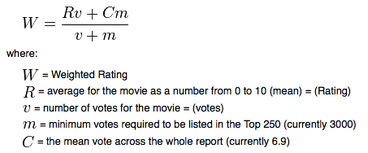Here’s one example of how to get the weighted averages:

``````V = movies_clean['vote_count']
R = movies_clean['vote_average']
C = movies_clean['vote_average'].mean()
m = movies_clean['vote_count'].quantile(0.70)

movies_clean['weighted_average'] = (V/(V+m) * R) + (m/(m+V) * C)``````

I selected _0.70 _as my argument for _quantile() _to indicate that I was concerned only with movies that received at least as many votes as 70% of the movies of our dataset. Selecting our value for m is a bit arbitrary, so do try some experimentation here.

#### Recommender Mk1:

Now we’re ready for our first recommendation system. Let’s recommend ten movies with the highest weighted average ratings:

``````import matplotlib.pyplot as plt
import seaborn as sns

wavg = movies_ranked.sort_values('weighted_average', ascending=False)

plt.figure(figsize=(16,6))

plt.xlim(6.75, 8.35)
plt.title('"Best" Movies by TMDB Votes', weight='bold')
plt.xlabel('Weighted Average Score', weight='bold')
plt.ylabel('Movie Title', weight='bold')

plt.savefig('best_movies.png')``````

And we get this lovely graph of our highest rated picks: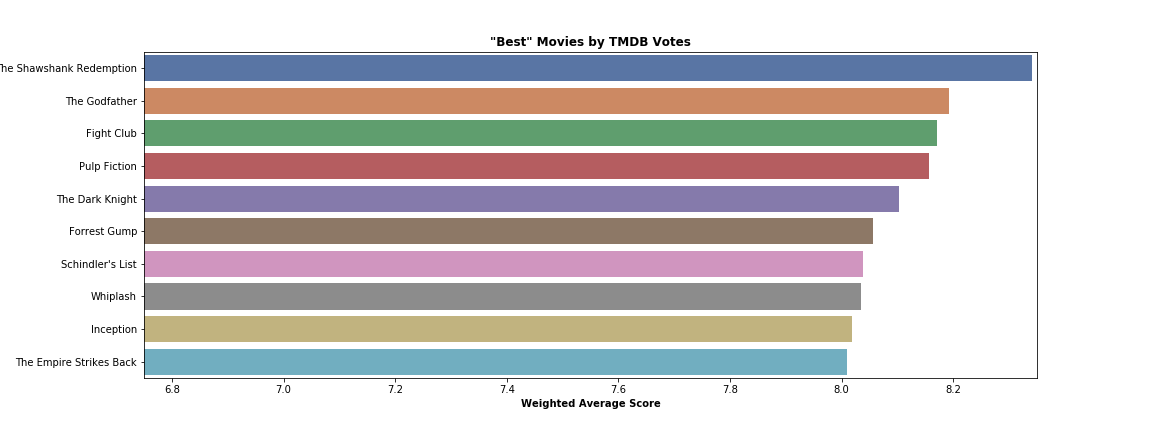We see that our inaugural system recommended some classics. But what if we want to recommend movies that are _popular _among TMDB users?

#### Recommender Mk2:

We can use the *popularity *feature of our data to recommend movies based on popularity instead:

``````popular = movies_ranked.sort_values('popularity', ascending=False)

plt.figure(figsize=(16,6))

plt.title('"Most Popular" Movies by TMDB Votes', weight='bold')
plt.xlabel('Popularity Score', weight='bold')
plt.ylabel('Movie Title', weight='bold')

plt.savefig('popular_movies.png')``````

And now we can see our recommendations based on popularity scores: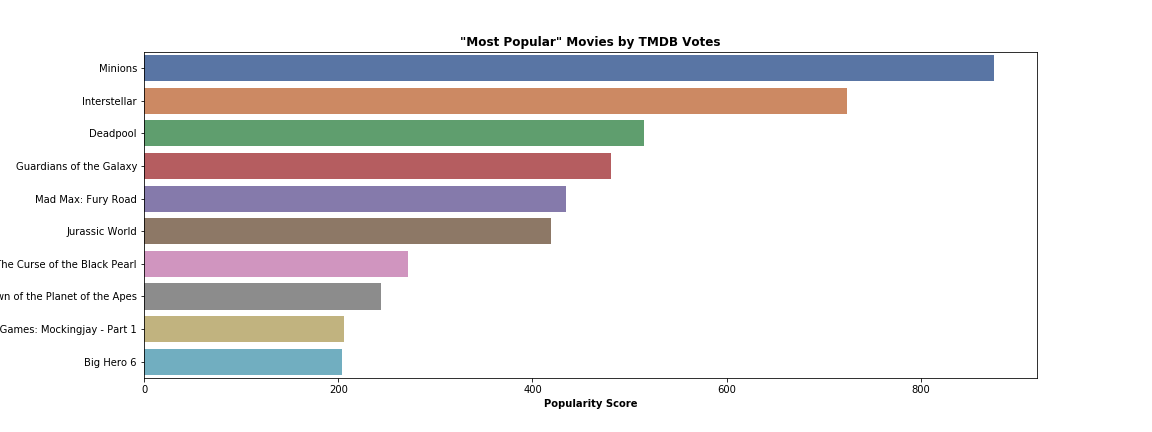Ah, just as we expected: a standout performance from Minions. Now what if we want to recommend movies based on their weighted average ratings _and_their popularity scores?

#### Recommender Mk3:

In order to avoid the colossal popularity score of Minions skewing our new scoring system, I normalized the values in both the *weighted_average *and *popularity *columns. I decided to go with a 50/50 split between the scaled weighted average rating and popularity scores, but again don’t be afraid to experiment with this split:

``````# My own recommender system
# half/half recommendation based on scaled weighted average & popularity score

from sklearn import preprocessing

min_max_scaler = preprocessing.MinMaxScaler()
movies_scaled = min_max_scaler.fit_transform(movies_clean[['weighted_average', 'popularity']])
movies_norm = pd.DataFrame(movies_scaled, columns=['weighted_average', 'popularity'])

movies_clean[['norm_weighted_average', 'norm_popularity']] = movies_norm

movies_clean['score'] = movies_clean['norm_weighted_average'] * 0.5 + movies_clean['norm_popularity'] * 0.5
movies_scored = movies_clean.sort_values(['score'], ascending=False)

Now that we have a new *score *column that takes into account a movie’s weighted average rating and it’s popularity score, we can see what movies our recommender system will offer us:

``````scored = movies_clean.sort_values('score', ascending=False)

plt.figure(figsize=(16,6))

#plt.xlim(3.55, 5.25)
plt.title('Best Rated & Most Popular Blend', weight='bold')
plt.xlabel('Score', weight='bold')
plt.ylabel('Movie Title', weight='bold')

plt.savefig('scored_movies.png')``````

And here are our recommendations based on my 50/50 split: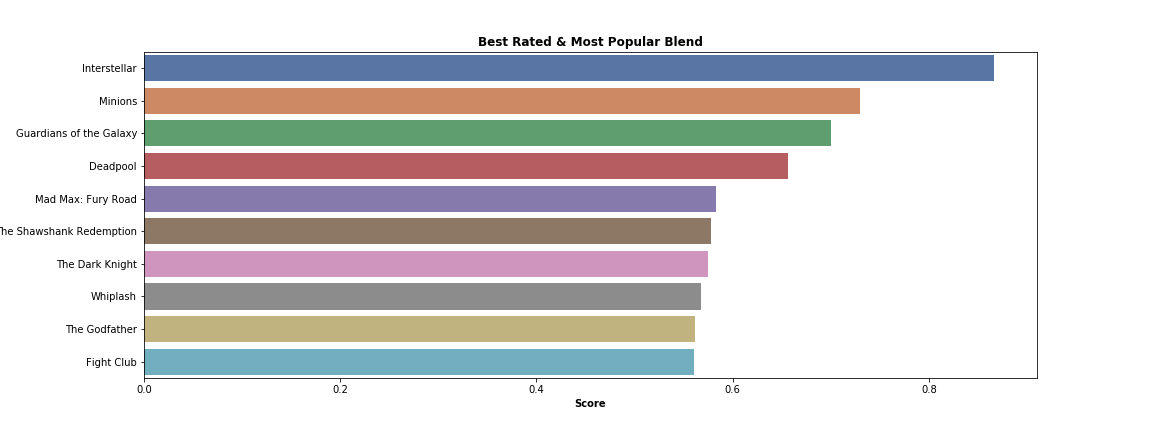These recommenders worked as intended, but we can certainly improve. Now we’ll have to turn to content-based filtering.

### Content-Based Filtering

So now we’re interested in using the characteristics of a movie in order to recommend other movies to the user. Again following Ibtesam’s example, we’ll now make recommendations based on the movie’s plot summaries given in the overview column. So if our user gives us a movie title, our goal is to recommend movies that share similar plot summaries.

#### Word Vectorization and TF-IDF

Before we can begin any analysis on the plot summaries, we’ll have to convert our text in the overview column to word vectors, and we’ll have to fit a TF-IDF on overview as well:

``````from sklearn.feature_extraction.text import TfidfVectorizer

# Using Abhishek Thakur's arguments for TF-IDF
tfv = TfidfVectorizer(min_df=3,  max_features=None,
strip_accents='unicode', analyzer='word',token_pattern=r'\w{1,}',
ngram_range=(1, 3), use_idf=1,smooth_idf=1,sublinear_tf=1,
stop_words = 'english')

# Filling NaNs with empty string
movies_clean['overview'] = movies_clean['overview'].fillna('')

# Fitting the TF-IDF on the 'overview' text
tfv_matrix = tfv.fit_transform(movies_clean['overview'])

tfv_matrix.shape``````

And we receive the following output:

• (4803, 10417)

So about 10,000 unique words were used in the plot summaries to describe our 5,000 movies (note that this figure is smaller than Ibtesam’s because I increased the minimum word frequency to 3 with min_df=3). If you’re interested in more, I talk about TF-IDF in this article, too.

#### Calculating Similarity Scores

Now that we have a matrix of our words, we can begin calculating similarity scores. This metric will help us pick out movies with plot summaries similar to the movie submitted by the user. Ibtesam opted for the linear kernel, but I wanted to experiment with the sigmoid kernel for fun. Luckily, I arrived at similar results:

``````from sklearn.metrics.pairwise import sigmoid_kernel

# Compute the sigmoid kernel
sig = sigmoid_kernel(tfv_matrix, tfv_matrix)

# Reverse mapping of indices and movie titles
indices = pd.Series(movies_clean.index, index=movies_clean['original_title']).drop_duplicates()

# Credit to Ibtesam Ahmed for the skeleton code
def give_rec(title, sig=sig):
# Get the index corresponding to original_title
idx = indices[title]

# Get the pairwsie similarity scores
sig_scores = list(enumerate(sig[idx]))

# Sort the movies
sig_scores = sorted(sig_scores, key=lambda x: x, reverse=True)

# Scores of the 10 most similar movies
sig_scores = sig_scores[1:11]

# Movie indices
movie_indices = [i for i in sig_scores]

# Top 10 most similar movies
return movies_clean['original_title'].iloc[movie_indices]
``````

So now that we’ve constructed our content-based filtering system, let’s test it out with timeless favorite, Spy Kids:

``````# Testing our content-based recommendation system with the seminal film Spy Kids
give_rec('Spy Kids')``````

And here are our recommendations per the content-based filtering system: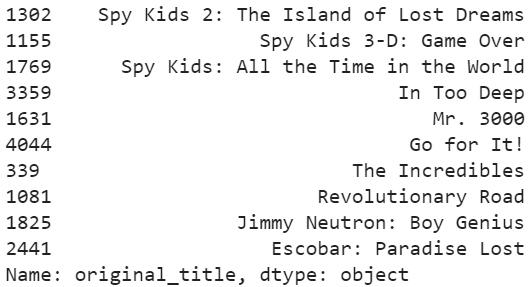So our recommendation system gave us some picks related to Spy Kids, but a few missteps such as _In Too Deep _and _Escobar: Paradise Lost _slipped in.

#### Limitations

Based on our results above, we can see that our content-based filtering system has some limitations:

1. Our recommender picked some movies that would probably be deemed inappropriate by a user searching for titles related to _Spy Kids. _To improve our system, we could consider replacing TF-IDF with word counts, and we could also explore other similarity scores.
2. Our system only considers the plot summaries of each movie as it stands now. If we, like Ibtesam, consider other features such as the cast members, the director, and genre, we’ll probably improve in finding related movies.
3. Our current system only recommends movies based on similarities in characteristics. So our recommender is missing movies in other genres that the user might enjoy. We’d need to try collaborative filtering to solve this, but our dataset didn’t include user information.

#### Summary

To sum up, we covered the following:

What recommender systems are, how they work, and some of the different types How to implement very basic recommender systems based on weighted average ratings, popularity, and a blend of the two How to create a content-based filtering system and how to recognize the limitations of content-based recommendations alone

## Data Science Course in Dallas

Become a data analysis expert using the R programming language in this [data science](https://360digitmg.com/usa/data-science-using-python-and-r-programming-in-dallas "data science") certification training in Dallas, TX. You will master data...

## Python For Data Science - How to use Data Science with Python

This Edureka video on 'Python For Data Science - How to use Data Science with Python - Data Science using Python ' will help you understand how we can use python for data science along with various use cases. What is Data Science? Why Python? Python Libraries For Data Science. Roadmap To Data Science With Python. Data Science Jobs and Salary Trends

## Data Science with Python explained

An overview of using Python for data science including Numpy, Scipy, pandas, Scikit-Learn, XGBoost, TensorFlow and Keras.